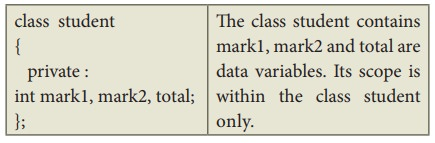Home | | Computer Science 11th std | Scope Rules of Variables

# Scope Rules of Variables

Scope refers to the accessibility of a variable. There are four types of scopes in C++.

Scope Rules of Variables

Scope refers to the accessibility of a variable. There are four types of scopes in C++.

They are: Local scope, Function scope, File scope and Class scope.

## Introduction

A scope is a region or life of the variable and broadly speaking there are three places, where variables can be declared,

• Inside a block which is called local variables.

• Inside a function is called function variables.

• Outside of all functions which is called global variables.

• Inside a class is called class variable or data members.

## Local Scope:

• A local variable is defined within a block.A block of code begins and ends with curly braces { }.

• The scope of a local variable is the block in which it is defined.

• A local variable cannot be accessed from outside the block of its declaration.

• A local variable is created upon entry into its block and destroyed upon exit.

### Program 11.28 (a)

//Demo to test Local Scope//

#include<iostream>s

using namespace std;

int main ( )

{

int a, b ;

a = 10;

b = 20;

if (a > b)

{

int temp; //local to this if block//

temp = a;

a = b;

b = temp;

}

cout <<"\n Descending order .... \n";

cout <<a <<"\t"<<b;

return(0);

}

### Output:

Descending order ….

10  20

### Program 11.28 (b)

//Demo to test Local Scope//

#include<iostream>s

using namespace std;

int main ( )

{

int a, b ;

a = 10;

b = 20;

if (a > b)

{

int temp; //local to this if block//

temp = a;

a = b;

b = temp;

}

cout <<temp;

return(0);

}

### In function 'int main()':

[Error] 'temp' was not declared in this scope

On compilation the Program 11.28(b), the compiler prompts an error message: The variable temp is not accessible. Because the life time of a local variable is the life time of a block in its state of execution. Local variables die when its block execution is completed.

## Function Scope:

• The scope of variables declared within a function is extended to the function block, and all sub-blocks therein.

• The life time of a function scope variable, is the life time of the function block. The scope of formal parameters is function scope.

### Program 11.29 (a)

//Demo to test Function Scope//

#include<iostream>

using namespace std;

{

int m=x+y; //'m' declared within function add()//

cout<<"\nThe Sum = "<<m;

}

int main ( )

{

int a, b ;

a = 10;

b = 20;

return(0);

}

### Program 11.29 (b)

//Demo to test Function Scope//

#include<iostream>

using namespace std;

{

int m=x+y; //'m' declared within function add()//

cout<<"\nThe Sum = "<<m;

}

int main ( )

{

int a, b ;

a = 10;

b = 20;

cout<<m; //'m' declared within function add()//

return(0);

}

### Output:

The Sum = 30

Note : In function 'int main()':

[Error] 'm' was not declared in this scope

## File Scope:

• A variable declared above all blocks and functions (including main ( ) ) has the scope of a file. The life time of a file scope variable is the life time of a program.

• The file scope variable is also called as global variable.

### Program 11.30

//Demo to test File or global Scope//

#include<iostream>

using namespace std;

int file_var=20; //Declared within File//

{

int m=x+y+file_var;

cout<<"\n The Sum = "<<m;

}

int main ( )

{

int a, b ;

a = 10;

b = 20;

cout<<”\nThe File Variable = “<<file_var;

return(0);

}

### Output:

The Sum = 50

The File Variable =20

## Class Scope:

• A class is a new way of creating and implementing a user defined data type. Classes provide a method for packing together data of different types.

• Data members are the data variables that represent the features or properties of a class.Note: The class scope will be discussed later in chapter “Classes and Object”.

## Scope resolution operator

• The scope operator reveals the hidden scope of a variable. The scope resolution operator (::) is used for the following purposes.

• To access a Global variable when there is a Local variable with same name. An example using Scope Resolution Operator.

### Program 11.31

//Program to show that we can access a global variable

//using scope resolution operator :: when there is a local

//variable with same name //

#include<iostream>

using namespace std;

int x=45; // Global Variable x

int main()

{

int x = 10; // Local Variable x

cout << "\nValue of global x is " << ::x;

cout << "\nValue of local x is " << x;

return 0;

}

### Output:

Value of global x is 45

Value of local x is 10

Tags : Introduction, Example C++ Program , 11th Computer Science : Chapter 11 : Functions
Study Material, Lecturing Notes, Assignment, Reference, Wiki description explanation, brief detail
11th Computer Science : Chapter 11 : Functions : Scope Rules of Variables | Introduction, Example C++ Program